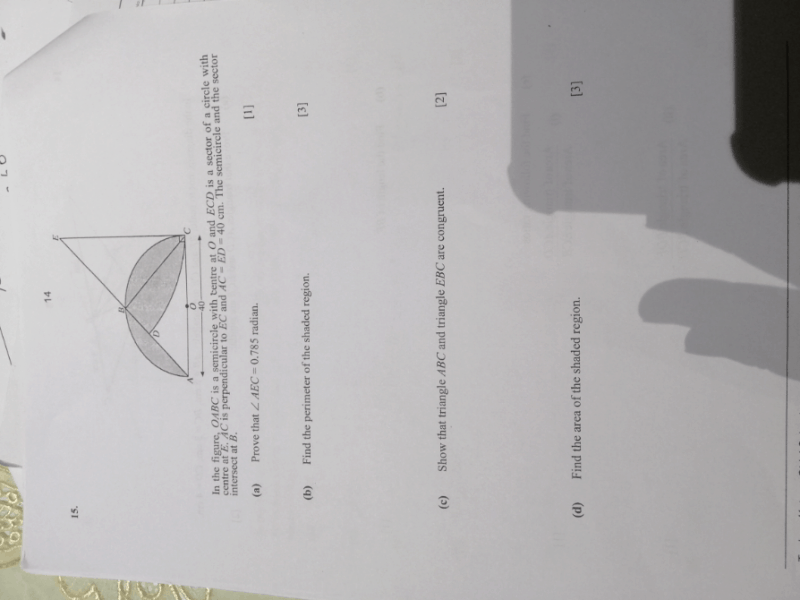# Questiona) AC = ED = 40 cm
Since ED and EC are both radius to a circle, ED = EC = 40 cm

Looking at △ ACE, since AC = EC = 40cm, and ∠ACE = 90°, △ ACE is a right isosceles △,
∠AEC + ∠EAC +90° = 180°
∠AEC + ∠EAC = 90°
Since ∠AEC = ∠EAC, ∠AEC = 45°

45° = (45 x π/180) rad

b) ABC = 1/2 x 2π(20)
= 20π cm

DC / 2π(40) = 45/360
⌒DC = 45/360 x 2π(40)
= 1/8 x 80
π
= 10π cm

(AE)2 = (AC)2 + (EC)2
(AE)2 = 402 + 402
AE = √3200
16.57 cm

= 20π + 10π + 16.57
= 30π + 16.57
110.82 cm

c) AC = EC = 40 cm (S)
∠BEC = ∠BAC = 45° (A)
BC is a common side of both △s (S)

∴ △ABC and △EBC are congruent by SAS congruence test

d) Area of ABC = 1/2 x 20 x 20
= 200 cm2
Area of semi-circle ABC = 1/2 x π x 20 x 20
= 200π cm2
Areas of ⌒AB and BC = (200π – 200) cm2

45/360 = Area of DEC / π(40)2
1/8 = Area of DEC / 1600π
Area of DEC = 1/8 x 1600π
= 200π cm2
Area of DBC = Area of DEC – Area of △EBC
Area of DBC = (200π – 200) cm2

Area of shaded region = Areas of ⌒AB and BC + Area of DBC
= (200π – 200) + (200π – 200)
= (400π – 400) cm2
856.64 cm2

0 Replies 0 Likes ✔Accepted Answer